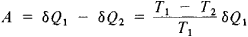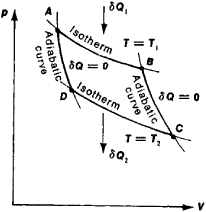# Carnot cycle

Also found in: Dictionary, Thesaurus, Wikipedia.
Related to Carnot cycle: Rankine cycle, Refrigeration cycle

## Carnot cycle

[kär′nō ‚si·kəl]
(thermodynamics)
A hypothetical cycle consisting of four reversible processes in succession: an isothermal expansion and heat addition, an isentropic expansion, an isothermal compression and heat rejection process, and an isentropic compression.

## Carnot Cycle

a reversible cyclic process in which heat is converted into work, or vice versa. It consists of two isothermal and two adiabatic processes that alternate.

The Carnot cycle was first examined by the French scientist N. L. S. Carnot (1824) as the ideal operating cycle of a heat engine. The conversion of heat into work is accompanied by the transfer of a certain amount of heat by the engine’s working substance from the hotter body (the heater) to the cooler.

The Carnot cycle proceeds as follows: at a temperature T1 the working substance (such as steam in a cylinder under a piston) is brought into contact with a heater that has a constant temperature T1 and isothermically acquires from it an amount of heat δQi (here the steam expands and performs work). In Figure 1 this process is represented by the segment AB of the isotherm. The working substance, expanding adiabatically (along the adiabatic curve BC ), then cools to temperature T2. At this temperature, as it becomes isothermically compressed (segment CD), it gives off a quantity of heat δQ2 to the cooler with temperature T2. The cycle ends with an adiabatic process (DA in Figure 1) that returns the working substance to its initial thermodynamic state. When the temperature difference (T1T2) between the heater and cooler is constant, the working substance performs the following amount of work in one cycle:This work is numerically equal to the area ABCD (Figure 1) bounded by the segments of the isotherms and adiabatic curves forming Carnot’s cycle.Figure 1. The Carnot cycle in a (p, V)(´Q1) quantity of heat received by the working substance from the heater, (´Q2) quantity of heat released by the working substance to the cooler. The area ABCD is numerically equal to the wor^k performed in the cycle.

The Carnot cycle is reversible and can be carried out in the reverse sequence (in the direction ADCBA). Here the quantity of heat ´Q2 is collected from the cooler and, together with the lost work ´A (which has been converted into heat), is transferred to the heater. A heat engine operates in this mode as an ideal cooler.

The Carnot cycle has the highest efficiency η = ´A/´Q1 = (T1T2)/T1 of all possible cycles accomplished in the same temperature interval (T1T2 ). In this sense the efficiency of the Carnot cycle serves as a measure of the efficiency of other working cycles.

Historically, the Carnot cycle played an important role in the development of thermodynamics and heat engineering. It was used to prove the equivalency of the formulations of the second law of thermodynamics given by R. Clausius and W. Thomson

(Lord Kelvin), to determine the absolute temperature scale, and frequently also to derive various thermodynamic relations (such as the Clausius-Clapeyron equation)

### REFERENCES

Fermi, E. Termodinamika. Kharkov, 1969. (Translated from English.)
Putilov, K. A. Termodinamika. Moscow, 1971.

## Carnot cycle

A hypothetical thermodynamic cycle used as a standard of comparison for actual cycles. The Carnot cycle shows that, even under ideal conditions, a heat engine cannot convert all the heat energy supplied to it into mechanical energy; some of the heat energy must be rejected.

In a Carnot cycle, an engine accepts heat energy from a high-temperature source, or hot body, converts part of the received energy into mechanical (or electrical) work, and rejects the remainder to a low-temperature sink, or cold body. The greater the temperature difference between the source and sink, the greater the efficiency of the heat engine.

The Carnot cycle (see illustration) consists first of an isentropic compression, then an isothermal heat addition, followed by an isentropic expansion, and concludes with an isothermal heat rejection process. In short, the processes are compression, addition of heat, expansion, and rejection of heat, all in a qualified and definite manner. The net effect of the cycle is that heat is added at a constant high temperature, somewhat less heat is rejected at a constant low temperature, and the algebraic sum of these heat quantities is equal to the work done by the cycle.

A Carnot cycle consists entirely of reversible processes; thus it can theoretically operate to withdraw heat from a cold body and to discharge that heat to a hot body. To do so, the cycle requires work input from its surroundings. The heat equivalent of this work input is also discharged to the hot body. Just as the Carnot cycle provides the highest efficiency for a power cycle operating between two fixed temperatures, so does the reversed Carnot cycle provide the best coefficient of performance for a device pumping heat from a low temperature to a higher one. See Heat pump, Refrigeration cycle

Good as the ideal Carnot cycle may be, there are serious difficulties that emerge when one wishes to make an actual Carnot engine. The necessarily high peak pressures and temperatures limit the practical thermal efficiency that an actual engine can achieve. Although the Carnot cycle is independent of the working substance, and hence is applicable to a vapor cycle, the difficulty of efficiently compressing a vapor-liquid mixture renders the cycle impractical. See Power plant, Thermodynamic cycle, Thermodynamic principles

## Carnot cycle

A name given to the cycle of operations of an internal combustion engine that would take place in an ideally perfect engine in which friction is nonexistent and heat losses are totally absent. A theoretical cycle derived by Nicoles Leonhard Sadi Carnot, who enunciated it in 1874. The four events that occur in a Carnot cycle are (i) an isothermal expansion, (ii) followed by an adiabatic expansion, (iii) an isothermal compression, and (iv) to an adiabatic compression. The cycle is depicted in the illustration.
References in periodicals archive ?
the ratio of the heat pumped to the work input, the idealized Carnot cycle represents the thermodynamic maximum COP for pumping heat from a low-temperature heat source to a higher temperature heat sink.
He devised an ingenious type of Carnot cycle in which a series of three chemical reactions were arranged in a cycle.
It gives the ratio of chiller actual COP, defined in Equation 5, to the ideal efficiency of the reverse Carnot cycle operating between the same saturated temperatures as the actual chillers, defined in Equation 6.
Thus, it can be easily calculated that the maximum theoretical efficiency of a Carnot cycle between [A.
These and other losses permit gas-compression cooling technology to reach only 50 percent of the theoretical maximum efficiency of the Carnot cycle.
The Carnot cycle is the ideal refrigeration cycle operating between two fixed temperatures with the highest system efficiency.
The ICE's efficiency is limited by the Carnot cycle and has a theoretical maximum efficiency of 40 to 50 percent.
The Rankine cycle uses the phase change of a fluid from liquid to gas to replicate the Carnot cycle as closely as possible.
Carnot cycles (using only the concepts of heat and work) were originally conceived prior to the concept of exergy.

Site: Follow: Share:
Open / Close